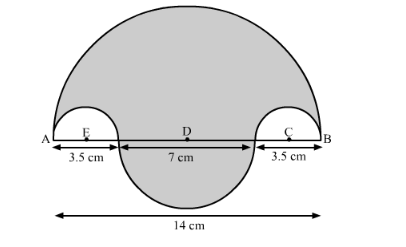# In the following figure, the boundary of the shaded region consistsQuestion:

In the following figure, the boundary of the shaded region consists of four semi-circular arcs, the smallest two being equal. If the diameter of the largest is 14 cm and of the smallest is 3.5 cm, find

(i) the length of the boundary.
(ii) the area of the shaded region.Solution:

(i) We will first find the length of the boundary.

Length of the boundaryperimeter of semi-circle with diameter AB + boundary of semi-circle with diameter 7 cm

Length of the boundary $=(\pi \times 7+\pi \times 3.5)+\pi \times 1.75+\pi \times 1.75$

$\therefore$ Length of the boundary $=14 \pi$

$\therefore$ Length of the boundary $=44$

Therefore, length of the boundary is $44 \mathrm{~cm}$.

Now we will find the area of the shaded region as shown below,

Area of the shaded region = Area of the semi-circle with AB as a diameter − area of the semi-circle with radius AE − area of the semi-circle with radius BC + area of the semi-circle with diameter 7 cm.

$\therefore$ Area of the shaded region $=\frac{\pi \times 7 \times 7}{2}-\frac{\pi \times 1.75 \times 1.75}{2}-\frac{\pi \times 1.75 \times 1.75}{2}+\frac{\pi \times 3.5 \times 3.5}{2}$

Area of the shaded region $=\frac{49 \pi}{2}-3.0625 \pi+\frac{12.25 \pi}{2}$

$\therefore$ Area of the shaded region $=\frac{61.25 \pi}{2}-3.0625 \pi$

$\therefore$ Area of the shaded region $=\frac{61.25 \pi-6.125 \pi}{2}$

$\therefore$ Area of the shaded region $=55.125 \times \frac{22}{7} \times \frac{1}{2}$

$\therefore$ Area of the shaded region $=7.875 \times 11$

$\therefore$ Area of the shaded region $=86.625$

Therefore, area of the shaded region is $86.625 \mathrm{~cm}^{2}$.